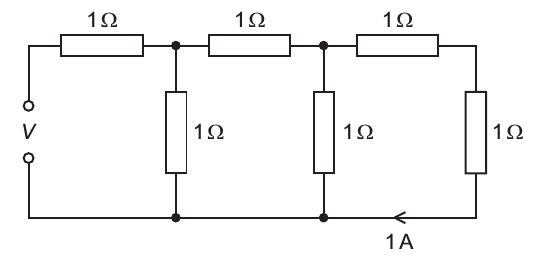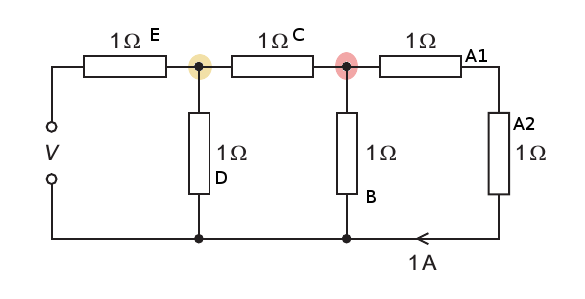37 A network of resistors, each of resistance 1 Ω, is connected as shown.The current passing through the end resistor is 1 A. What is the potential difference (p.d.) V across the input terminals?
A: 2V
B: 5V
C: 8V
D: 13 V

We know the resistances, so we can calculate the total resistance of the circuit.

```Calculate the combined resistance of A1+A2 and B: 1/(1+1/2) = 2/3
Calculate the combined resistance of (A1+A2, and B), C and D = 1/(1+1/(2/3+1)) = .625
Finally, adding the resistance of E to it = 1.625```Now the current through the rightmost two resistors (A1 and A2) is 1A hence the total PD across them is 2V. Since they are connected in parallel with another 1 ohm resistor (labelled B), the PD across B is 2V as well, (all thanks to Kirchoff’s second law.) So it must have a current of 2A flowing through it because V=IR.

According to Kirchoff’s first law, the total current entering the junction (we are looking at the one highlighted in red) must equal the total current leaving the junction. So we add the two currents to get the current across resistor C i.e. 3A, so the potential difference across it is 3V.

The 3 resistors (A1,A2 and B) can be interpreted as a single resistor (call it X) connected in series with resistor C and having a potential of 2V across it. Adding the 2 potentials of X and C gives us 5, which is also equal to the potential across resistor D which is connected in parallel with C+X. The current through D must therefore be 5A. Looking at the junction highlighted in yellow, we can see that the current entering the junction and hence the current across the leftmost resistor(labelled E) is equal to the sum of the current across D and the current across C+X: 5+3=8A.

Total resistance(1.625) multiplied by this value of current(8A) equals the EMF i.e. 1.625*8=13V.